Question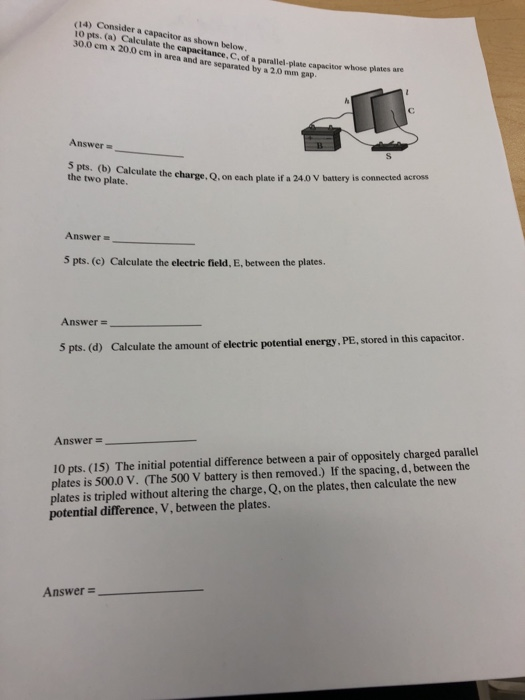We need at least 10 more requests to produce the answer.

0 / 10 have requested this problem solution

The more requests, the faster the answer.

All students who have requested the answer will be notified once they are available.

#### Earn Coins

Coins can be redeemed for fabulous gifts.

Similar Homework Help Questions
• ### The potential difference between a pair of oppositely charged parallel plates is 430 V. (a) If...

The potential difference between a pair of oppositely charged parallel plates is 430 V. (a) If the spacing between the plates is doubled without altering the charge on the plates, what is the new potential difference between the plates? V (b) If the plate spacing is tripled while the potential difference between the plates is kept constant, what is the ratio of the final charge on one of the plates to the original charge? (Qfinal / Qinitial)

• ### 1. (8 points total) A parallel plate capacitor whose plates have area A and plate separation d is...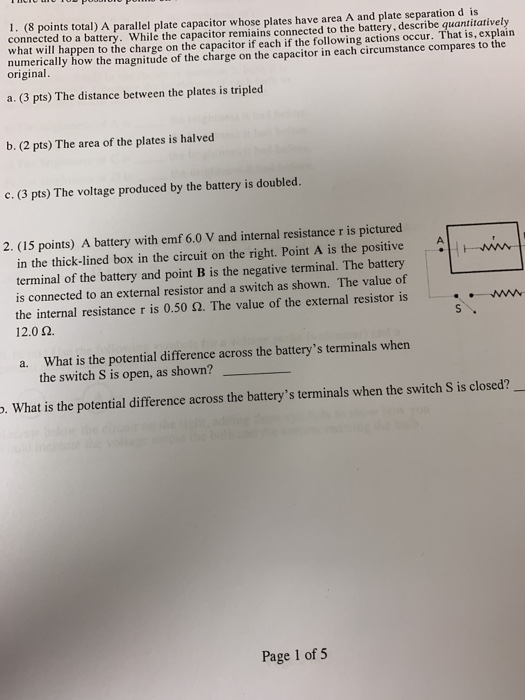i need help with this page??? 1. (8 points total) A parallel plate capacitor whose plates have area A and plate separation d is connected to a battery. While the capacitor remiains connected to the battery, describe quantitatively what will happen to the charge on the capacitor if each if the following actions occur. That is, explain numerically how the magnitude of the charge on the capacitor in each circumstance compares to the original a. (3 pts) The distance between...

• ### A parallel-plate capacitor with plate area 4.60 cm2 and air-gap separation 0.78 mm is connected to...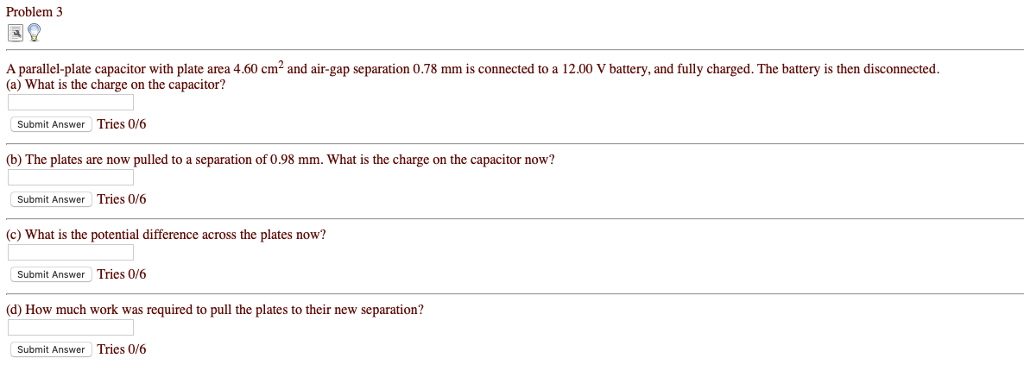A parallel-plate capacitor with plate area 4.60 cm2 and air-gap separation 0.78 mm is connected to a 12.00 V battery, and fully charged. The battery is then disconnected. (a) What is the charge on the capacitor? (b) The plates are now pulled to a separation of 0.98 mm. What is the charge on the capacitor now? (c) What is the potential difference across the plates now? (d) How much work was required to pull the plates to their new separation?...

• ### Problem 3 A parallel-plate capacitor with plate area 5.00 cm and air-gap separation 0.29 mm is...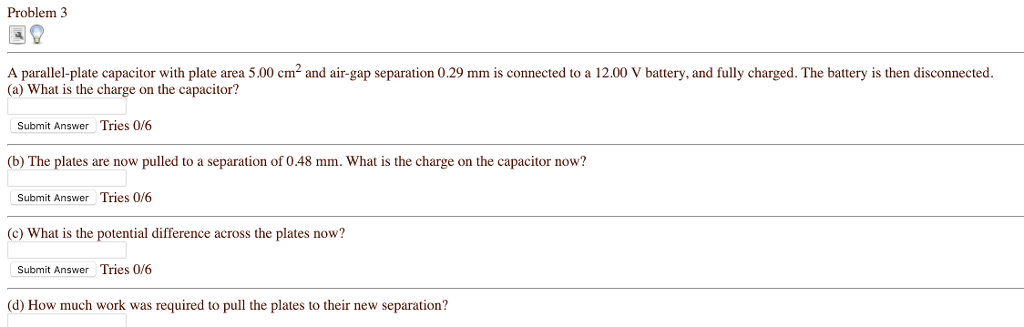Problem 3 A parallel-plate capacitor with plate area 5.00 cm and air-gap separation 0.29 mm is connected to a 12.00 V battery, and fully charged. The battery is then disconnected. (a) What is the charge on the capacitor? Submit Answer Tries 0/6 (b) The plates are now pulled to a separation of 0.48 mm. What is the charge on the capacitor now? Submit Answer Tries 0/6 (c) What is the potential difference across the plates now? Submit Answer Tries 0/6...

• ### A parallel-plate capacitor with plate area 4.0cm^2 and air-gap separation 0.50❝mm is connected to a 9.0-V...

A parallel-plate capacitor with plate area 4.0cm^2 and air-gap separation 0.50❝mm is connected to a 9.0-V battery, and fully charged. The battery is then disconnected. What is the charge on the capacitor? The plates are now pulled to a separation of 0.75?mm. What is the charge on the capacitor now? What is the potential difference between the plates now? How much work was required to pull the plates to their new separation?

• ### 85. A parallel-plate capacitor with plate area 3.0 cm2 and air- gap separation 0.50 mm is...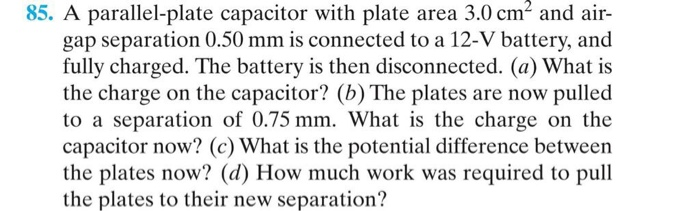85. A parallel-plate capacitor with plate area 3.0 cm2 and air- gap separation 0.50 mm is connected to a 12-V battery, and fully charged. The battery is then disconnected. (a) What is the charge on the capacitor? (b) The plates are now pulled to a separation of 0.75 mm. What is the charge on the capacitor now? (c) What is the potential difference between the plates now? (d) How much work was required to pull the plates to their new...

• ### Problem3 A parallel-plate capacitor with plate area 8.30 cm2 and air-gap separation 0.57 mm is connected...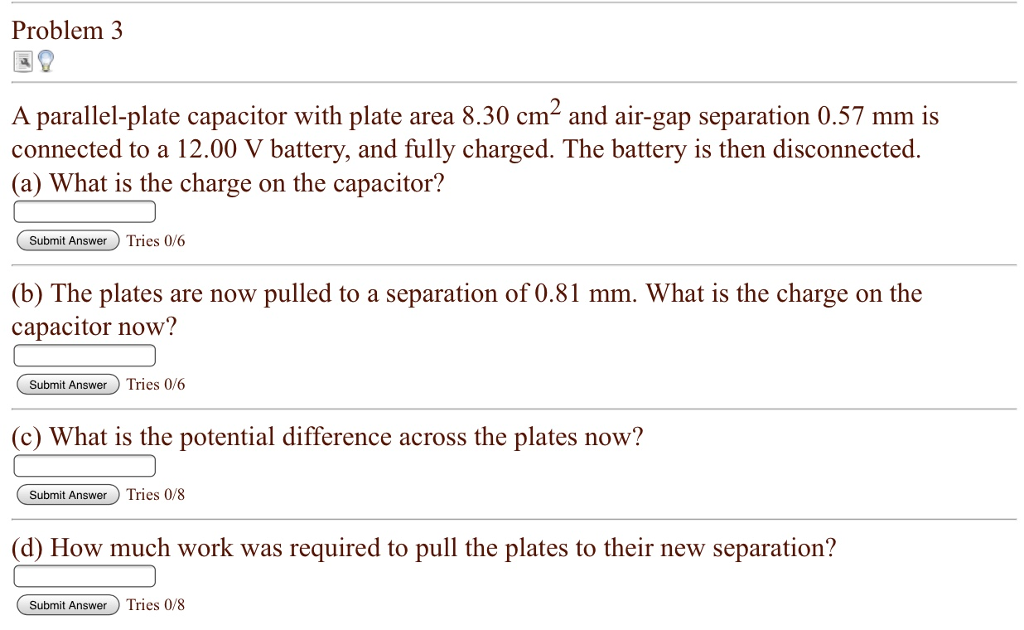Problem3 A parallel-plate capacitor with plate area 8.30 cm2 and air-gap separation 0.57 mm is connected to a 12.00 V battery, and fully charged. The battery is then disconnected. a) What is the charge on the capacitor? Submit AnswerTries 0/6 atery and fully charged. The (b) The plates are now pulled to a separation of 0.81 mm. What is the charge on the capacitor now? Subnmit AnswerTries 0/6 (c) What is the potential difference across the plates now? Submit Answer...

• ### HELP PLS!! Problem 3 A parallel-plate capacitor with plate area 8.60 cm2 and air-gap separation 0.43...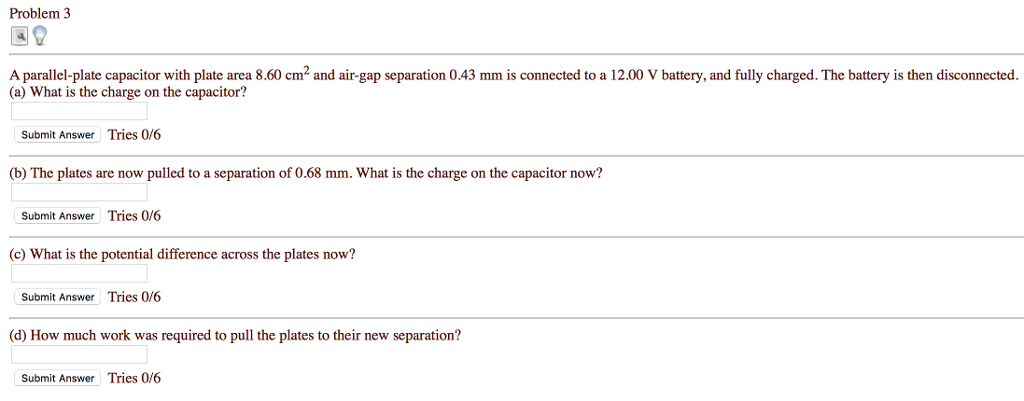HELP PLS!! Problem 3 A parallel-plate capacitor with plate area 8.60 cm2 and air-gap separation 0.43 mm is connected to a 12.00 V battery, and fully charged. The battery is then disconnected. (a) What is the charge on the capacitor? Submit Answer Tries 0/6 (b) The plates are now pulled to a separation of 0.68 mm. What is the charge on the capacitor now? Submit Answer Tries 0/6 c) What is the potential difference across the plates now? Submit Answer...

• ### The charged capacitor from problem 1 is now disconnected from the battery. The isolated capacitor has...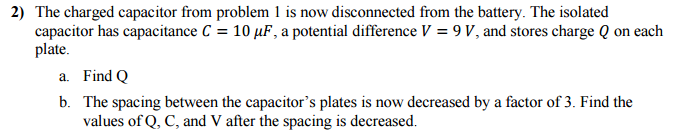The charged capacitor from problem 1 is now disconnected from the battery. The isolated capacitor has capacitance C = 10 mu F, a potential difference V = 9 V, and stores charge Q on each plate. a. Find Q b. The spacing between the capacitor's plates is now decreased by a factor of 3. Find the values of Q, C, and V after the spacing is decreased.

• ### A parallel-plate capacitor has plates of area 400 cm2 and is connected across the terminals of...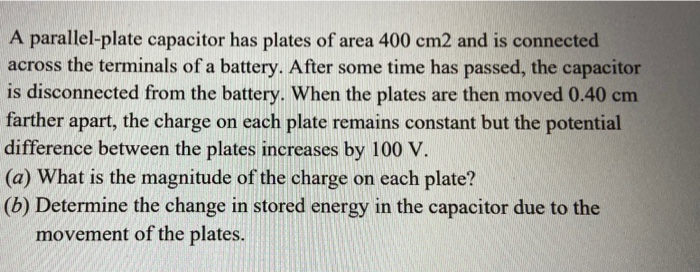A parallel-plate capacitor has plates of area 400 cm2 and is connected across the terminals of a battery. After some time has passed, the capacitor is disconnected from the battery. When the plates are then moved 0.40 cm farther apart, the charge on each plate remains constant but the potential difference between the plates increases by 100 V. (a) What is the magnitude of the charge on each plate? (5) Determine the change in stored energy in the capacitor due...